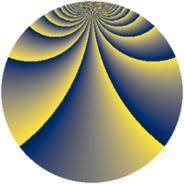# Properties

 Label 1323.2.cfLevel $1323$ Weight $2$ Character orbit 1323.cf Rep. character $\chi_{1323}(47,\cdot)$ Character field $\Q(\zeta_{126})$ Dimension $5976$ Sturm bound $336$

# Related objects

## Defining parameters

 Level: $$N$$ $$=$$ $$1323 = 3^{3} \cdot 7^{2}$$ Weight: $$k$$ $$=$$ $$2$$ Character orbit: $$[\chi]$$ $$=$$ 1323.cf (of order $$126$$ and degree $$36$$) Character conductor: $$\operatorname{cond}(\chi)$$ $$=$$ $$1323$$ Character field: $$\Q(\zeta_{126})$$ Sturm bound: $$336$$

## Dimensions

The following table gives the dimensions of various subspaces of $$M_{2}(1323, [\chi])$$.

Total New Old
Modular forms 6120 6120 0
Cusp forms 5976 5976 0
Eisenstein series 144 144 0

## Trace form

 $$5976q - 39q^{2} - 33q^{3} - 39q^{4} - 33q^{5} + 24q^{6} - 36q^{7} - 45q^{8} - 39q^{9} + O(q^{10})$$ $$5976q - 39q^{2} - 33q^{3} - 39q^{4} - 33q^{5} + 24q^{6} - 36q^{7} - 45q^{8} - 39q^{9} - 12q^{10} - 39q^{11} - 33q^{12} - 42q^{13} - 210q^{14} - 30q^{15} - 27q^{16} - 54q^{17} - 18q^{18} - 54q^{19} - 24q^{20} - 57q^{21} - 30q^{22} - 111q^{23} - 6q^{24} - 39q^{25} - 42q^{27} - 72q^{28} - 174q^{29} - 18q^{30} - 54q^{31} - 51q^{32} - 33q^{33} - 60q^{34} - 54q^{35} - 294q^{36} - 15q^{37} - 75q^{38} - 9q^{39} - 33q^{40} - 42q^{41} + 267q^{42} - 30q^{43} - 63q^{44} + 21q^{45} - 15q^{46} + 39q^{47} - 72q^{49} - 63q^{50} - 75q^{51} - 33q^{52} + 270q^{53} - 159q^{54} - 84q^{55} + 9q^{56} - 30q^{57} - 39q^{58} - 33q^{59} + 33q^{60} + 21q^{61} - 162q^{62} - 9q^{63} - 471q^{64} - 18q^{65} - 105q^{66} - 18q^{67} - 207q^{68} - 438q^{69} - 27q^{70} - 45q^{71} - 51q^{72} - 21q^{73} - 51q^{74} + 219q^{75} - 90q^{76} + 15q^{77} - 48q^{78} - 18q^{79} + 72q^{80} - 39q^{81} - 66q^{82} - 132q^{83} - 324q^{84} - 15q^{85} - 93q^{86} - 33q^{87} - 87q^{88} - 54q^{89} + 39q^{90} + 90q^{92} - 219q^{93} - 33q^{94} - 150q^{95} - 789q^{96} + 117q^{98} + O(q^{100})$$

## Decomposition of $$S_{2}^{\mathrm{new}}(1323, [\chi])$$ into newform subspaces

The newforms in this space have not yet been added to the LMFDB.